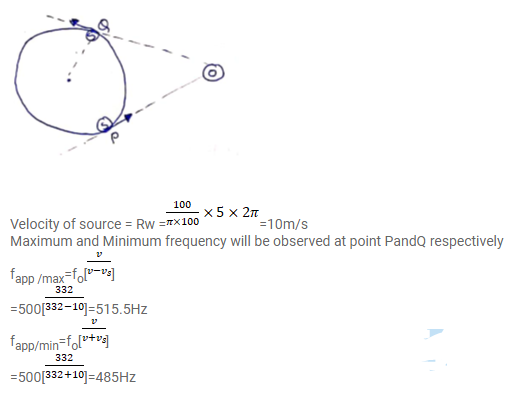# A small source of sound vibrating at frequencyQuestion:

A small source of sound vibrating at frequency $500 \mathrm{~Hz}$ is rotated in a circle of radius $100 / \pi \mathrm{cm}$ at a constant angular speed of $5.0$ revolutions per second. A listener situation situates himself in the plane of the circle. Find the minimum and the maximum frequency of the sound observed. Speed of sound in air $=332 \mathrm{~m} \mathrm{~s}^{-1}$.

Solution: